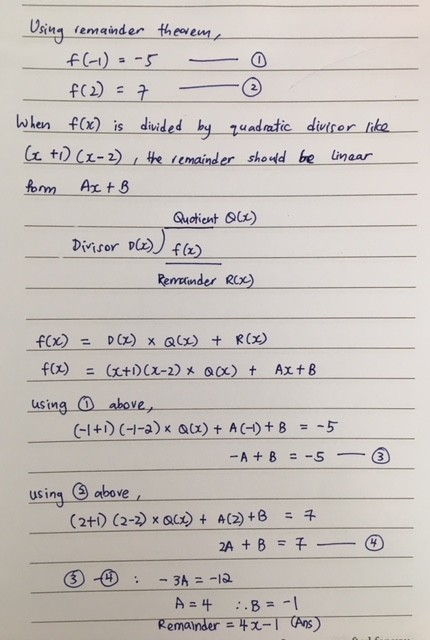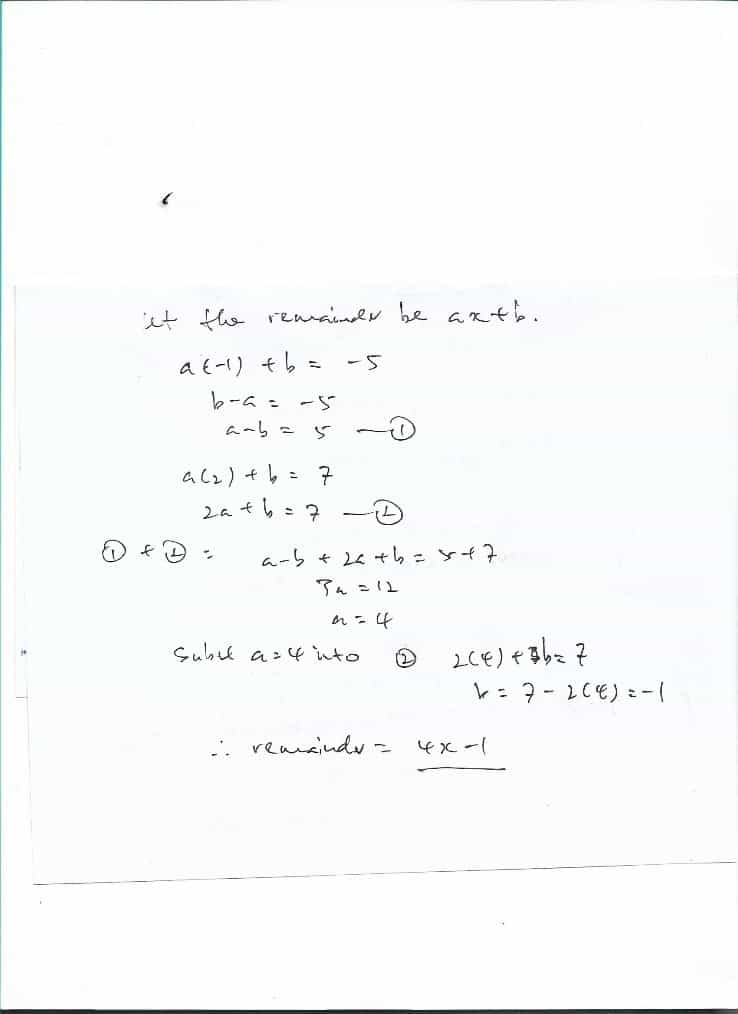# Question

The polynomial f(x) has a remainder of -5 when divided by (x+1) and a remainder of 7 when divided by (x-2). Find the remainder when f(x) is divided by (x+1)(x-2).